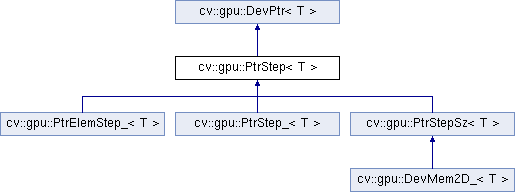OpenCV  2.4.13.3 Open Source Computer Visioncv::gpu::PtrStep< T > Struct Template Reference

`#include <cuda_devptrs.hpp>`

Inheritance diagram for cv::gpu::PtrStep< T >:## Public Member Functions

PtrStep ()

PtrStep (T *data_, size_t step_)

T * ptr (int y=0)

const T * ptr (int y=0) const

T & operator() (int y, int x)

const T & operator() (int y, int x) constPublic Member Functions inherited from cv::gpu::DevPtr< T >
DevPtr ()

DevPtr (T *data_)

size_t elemSize () const

operator T* ()

operator const T * () const

## Public Attributes

size_t step
stride between two consecutive rows in bytes. Step is stored always and everywhere in bytes!!! More...Public Attributes inherited from cv::gpu::DevPtr< T >
T * data

## Additional Inherited MembersPublic Types inherited from cv::gpu::DevPtr< T >
enum  { elem_size = sizeof(elem_type) }

typedef T elem_type

typedef int index_type

## § PtrStep() [1/2]

template<typename T>
 cv::gpu::PtrStep< T >::PtrStep ( )
inline

## § PtrStep() [2/2]

template<typename T>
 cv::gpu::PtrStep< T >::PtrStep ( T * data_, size_t step_ )
inline

## § operator()() [1/2]

template<typename T>
 T& cv::gpu::PtrStep< T >::operator() ( int y, int x )
inline

## § operator()() [2/2]

template<typename T>
 const T& cv::gpu::PtrStep< T >::operator() ( int y, int x ) const
inline

## § ptr() [1/2]

template<typename T>
 T* cv::gpu::PtrStep< T >::ptr ( int y = `0` )
inline

## § ptr() [2/2]

template<typename T>
 const T* cv::gpu::PtrStep< T >::ptr ( int y = `0` ) const
inline

## § step

template<typename T>
 size_t cv::gpu::PtrStep< T >::step

stride between two consecutive rows in bytes. Step is stored always and everywhere in bytes!!!

The documentation for this struct was generated from the following file: